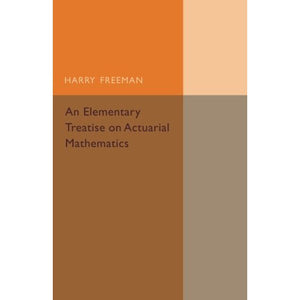># An Elementary Treatise on Actuarial Mathematics

• £18.29
• Save £19

Harry Freeman
Cambridge University Press, 5/26/2016
EAN 9781316611784, ISBN10: 1316611787

Paperback, 414 pages, 21.6 x 14.2 x 2.5 cm
Language: English

Originally published in 1931, this book was written to provide actuarial students with a guide to mathematics, with information on elementary trigonometry, finite differences, summation, differential and integral calculus, and probability. Examples are included throughout. This book will be of value to anyone with an interest in actuarial practice and its relationship with aspects of mathematics.

Introduction
Author's preface
Part I. Elementary Trigonometry
1. Definitions
Part II. Finite Differences
2. Definitions and fundamental formulae
3. Interpolation for equal intervals
4. Interpolation for unequal intervals
5. Central differences
6. Inverse interpolation
7. Summation
8. Miscellaneous theorems
Part III. Functions and Limits
9. Algebraic functions
Part IV. Differential Calculus
10. Definitions, standard forms, successive differentiation
11. Expansions
12. Maxima and minima
13. Miscellaneous theorems
Part V. Integral Calculus
14. Definitions and standard forms
15. More difficult integrals, integration by parts
16. Definite integrals, areas, miscellaneous theorems
17. Approximate integration
Part VI. Probability
18. Numerical definitions of probability
19. Mean value. The application of the calculus to the solution of questions in probability
Miscellaneous examples